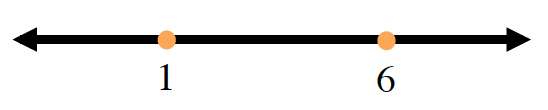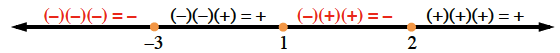### Home > PC > Chapter 7 > Lesson 7.2.3 > Problem7-68

7-68.

Solve the inequalities and express the solutions in both interval and inequality notation.

1. $x^2-7x\le-6$

Solve this as an equation. Being by setting one side equal to $0$.

$x^2−7x+6=0$

Factor and determine the "boundary points".

$(x−6)(x−1)=0$Test a point in each interval to see whether or not it makes the original inequality true.

1. $(x-2)(x-1)(x+3)<0$